# Assertions & Reason Type Questions: Real Numbers Notes | Study Mathematics (Maths) Class 10 - Class 10

## Class 10: Assertions & Reason Type Questions: Real Numbers Notes | Study Mathematics (Maths) Class 10 - Class 10

The document Assertions & Reason Type Questions: Real Numbers Notes | Study Mathematics (Maths) Class 10 - Class 10 is a part of the Class 10 Course Mathematics (Maths) Class 10.
All you need of Class 10 at this link: Class 10

Directions: In the following questions, a statement of assertion (A) is followed by a statement of reason (R). Mark the correct choice as:

Question 1:
Assertion : √x is an irrational number, where x is a prime number.
Reason : Square root of any prime number is an irrational number.

(a) Both assertion (A) and reason (R) are true and reason (R) is the correct explanation of assertion (A).
(b) Both assertion (A) and reason (R) are true but reason (R) is not the correct explanation of assertion (A).
(c) Assertion (A) is true but reason (R) is false.
(d) Assertion (A) is false but reason (R) is true.

As we know that square root of every prime number is an irrational number. So, both A and R are correct and R explains A.

Question 2:
Assertion :
The HCF of two numbers is 18 and their product is 3072. Then their LCM = 169.
Reason : If a, b are two positive integers, then HCF x LCM = a x b.
(a) Both assertion (A) and reason (R) are true and reason (R) is the correct explanation of assertion (A).
(b) Both assertion (A) and reason (R) are true but reason (R) is not the correct explanation of assertion (A).
(c) Assertion (A) is true but reason (R) is false.
(d) Assertion (A) is false but reason (R) is true.

Here reason is true [standard result] Assertion is false.
We know that for any two numbers, Product of the two numbers = HCF x LCM HCF x LCM = 18  x 169 = 3042 ≠ 3072

Question 3:
Assertion : 29/625 is a terminating decimal fraction.
Reason : If q = 2m x 5n where n, m are non-negative integers, then p/q is a terminating decimal fraction.
(a) Both assertion (A) and reason (R) are true and reason (R) is the correct explanation of assertion (A).
(b) Both assertion (A) and reason (R) are true but reason (R) is not the correct explanation of assertion (A).
(c) Assertion (A) is true but reason (R) is false.
(d) Assertion (A) is false but reason (R) is true.

Since the factors of the denominator 625 is of the form 20 x 54.
29/625 is a terminating decimal
Since, assertion follows from reason.

Question 4:
Assertion : When a positive integer a is divided by 4, the values of remainder can be 0, 1, 2 or 3.
Reason : According to Euclid’s Division Lemma a = bq + r , where 0 ≤ r < b and r is an integer.

(a) Both assertion (A) and reason (R) are true and reason (R) is the correct explanation of assertion (A).
(b) Both assertion (A) and reason (R) are true but reason (R) is not the correct explanation of assertion (A).
(c) Assertion (A) is true but reason (R) is false.
(d) Assertion (A) is false but reason (R) is true.

Given positive integers A and B, there exists unique integers ‘q’ and ‘r’ satisfying a = bq + r , where 0 ≤ r < b.

This is known as Euclid’s Division Lemma.
So, both A and R are correct and R explains A.

Question 5:
Assertion : 12n ends with the digit zero, where n is natural number.
Reason : Any number ends with digit zero, if its prime factor is of the form 2m x 5n, where m, n are natural numbers.

(a) Both assertion (A) and reason (R) are true and reason (R) is the correct explanation of assertion (A).
(b) Both assertion (A) and reason (R) are true but reason (R) is not the correct explanation of assertion (A).
(c) Assertion (A) is true but reason (R) is false.
(d) Assertion (A) is false but reason (R) is true.

12n = (2 x 2 x 3)n = 2n x 2n x 3n ,
Its prime factors do not contain 5n i.e., of the form 2m x 5n , where m, n are natural numbers.
Here assertion is incorrect but reason is correct.

Question 6:
Assertion : Denominator of 12.145. When expressed in the form p/q, q ≠ 0, is of the form 2m x 5n, where m, n are non-negative integers.
Reason : 12.145 is a terminating decimal fraction.
(a) Both assertion (A) and reason (R) are true and reason (R) is the correct explanation of assertion (A).
(b) Both assertion (A) and reason (R) are true but reason (R) is not the correct explanation of assertion (A).
(c) Assertion (A) is true but reason (R) is false.
(d) Assertion (A) is false but reason (R) is true.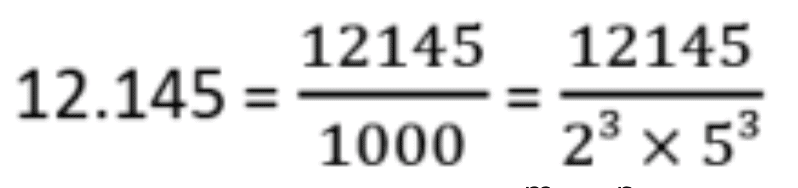Its denominator is of the form 2m x 5n, where m = 3, n = 3
Hence, assertion is true.
Since reason gives assertion

Question 7:
Assertion: 34.12345 is a terminating decimal fraction.
Reason: Denominator of 34.12345, when expressed in the form q/p , q ≠ 0, is of the form 2m x 5m , where m and n are non-negative integers.

(a) Both assertion (A) and reason (R) are true and reason (R) is the correct explanation of assertion (A).
(b) Both assertion (A) and reason (R) are true but reason (R) is not the correct explanation of assertion (A).
(c) Assertion (A) is true but reason (R) is false.
(d) Assertion (A) is false but reason (R) is true.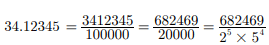Its denominator is of the form 2m x 5m , where m = 5 and n = 4 which are non-negative integers.

Question 8:
Assertion: 13/3125 is a terminating decimal fraction.
Reason: If q = 2m 5n where m, n are non-negative integers, then q/p is a terminating decimal fraction.

(a) Both assertion (A) and reason (R) are true and reason (R) is the correct explanation of assertion (A).
(b) Both assertion (A) and reason (R) are true but reason (R) is not the correct explanation of assertion (A).
(c) Assertion (A) is true but reason (R) is false.
(d) Assertion (A) is false but reason (R) is true.

We have 3125 = 55 = 55 x 20 = #

Since the factors of the denominator 3125 is of the form 20 x 55 , 13/3125 is a terminating decimal.

Question 9: Assertion: The HCF of two numbers is 5 and their product is 150, then their LCM is 30
Reason: For any two positive integers a and b, HCF (a, b) + LCM (a, b) = a x b.
(a) Both assertion (A) and reason (R) are true and reason (R) is the correct explanation of assertion (A).
(b) Both assertion (A) and reason (R) are true but reason (R) is not the correct explanation of assertion (A).
(c) Assertion (A) is true but reason (R) is false.
(d) Assertion (A) is false but reason (R) is true.

We have,
LCM(a, b) x HCF(a, b) = a x b
LCM x 5 = 150
LCM 5/150 = 30

Question 10:
Assertion (A): The decimal expansion of the rational number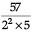will terminate after 2 decimal places.
Reason (R): The decimal expansion of the rational number 13/3125 will terminate after 3 decimal places.
(a) Both A and R are true and R is the correct explanation of A
(b) Both A and R are true but R is NOT the correct explanation of A
(c) A is true but R is false
(d) A is false and R is True

In case of assertion: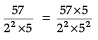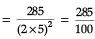= 2.85
So, it will terminate after 2 decimal places.
∴ Assertion is correct.
In case of Reason:
13/2125 = 13/55
[∵ prime factor of 3125 = 5 × 5 × 5 × 5]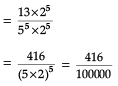= 0.00416.
So. it will terminate after 5 decimal places.
∴ Reason is incorrect.
Thus, assertion is correct but reason is incorrect.

Question 11:
Assertion (A): If HCF (336, 54) = 6, then LCM (336,54) = 3000.
Reason (R): The sum of exponents of prime factors in the prime factorisation of 196 is 4.

(a) Both A and R are true and R is the correct explanation of A
(b)
Both A and R are true but R is NOT the correct explanation of A
(c)
A is true but R is false
(d)
A is false and R is True

Let us consider the assertion,
∵ HCF × LCM = Product of numbers
∴ 6 × LCM = 336 × 54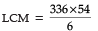= 3024
Thus, the assertion is incorrect:
Now, let us consider the reason:
Prime factors of 196 = 22 × 72

∴ The sum of exponents of prime factors = 2 + 2 = 4.

So, the reason is correct:
Thus, assertion is incorrect but reason is correct.

Question 12:
Assertion (A): The product of two consecutive positive integers is divisible by 2.
Reason (R): 13233343563715 is a composite number.

(a) Both A and R are true and R is the correct explanation of A
(b)
Both A and R are true but R is NOT the correct explanation of A
(c)
A is true but R is false
(d)
A is false and R is True

In case of assertion, Since, in the product of two consecutive positive integers, p = n(n + 1), one of n or (n + 1) is an even number.
Hence, the product of two consecutive positive integers is divisible by 2. So, it is correct.
Now, let us consider the reason:
Since, the given number ends in 5. It is a multiple of 5. Therefore, it is a composite number.
Thus, both assertion and reason are correct and reason is not the correct explanation for assertion.

Question 13:
Assertion (A): (7 × 13 × 11) + 11 and (7 × 6 × 5 × 4 × 3 × 2 × 1) + 3 have exactly composite numbers.
Reason (R): (3 × 12 × 101) + 4 is not a composite number.

(a) Both A and R are true and R is the correct explanation of A
(b)
Both A and R are true but R is NOT the correct explanation of A
(c)
A is true but R is false
(d)
A is false and R is True

Firstly consider the assertion,
Since (7 × 13 × 11) + 11 = 11 × (7 × 13 + 1) = 11 × (91 + 1)
= 11 × 92 ⇒ 11 × 2 × 2 × 23
and (7 × 6 × 5 × 4 × 3 × 2 × 1) + 3 = 3 (7 × 6 × 5 × 4 × 2 × 1 + 1)
= 3 × (1681) ⇒ 3 × 41 × 41
Given numbers have more than two prime factors.
So, both the numbers are composite. Hence, assertion is correct.
Now let us consider the reason: 3 × 12 × 101 + 4 = 4(3 × 3 × 101 + 1)

= 4(909 + 1)

= 4(910)

= 2 × 2 × 2 × 5 × 7 × 13
= a composite number
[∵ Product of more than two prime factors]
Thus, reason is not correct.
Thus, assertion is correct but reason is incorrect.

Question 14:
Assertion (A): HCF of two or more numbers = Product of the smallest power of each common prime factor, involved in the numbers.
Reason (R): The HCF of 12, 21 and 15 is 3.
(a) Both A and R are true and R is the correct explanation of A
(b) Both A and R are true but R is NOT the correct explanation of A
(c) A is true but R is false
(d) A is false and R is True

Let a, a2 and a3 be three numbers, then we have the smallest power of a1, a2 and a3 is 1. So, HCF is a.

Now, let us consider the reason:
Prime factors of 12 = 22 × 3
Prime factors of 21 = 3 × 7
Prime factors of 15 = 3 × 5
∴ HCF of 12, 21 and 15 = 3, which is a common prime factor.

Thus both assertion and reason are correct and reason is the correct explanation for assertion.

Question 15:
Assertion (A): The decimal expansion of 15/16000 is 0.09375.
Reason (R): The decimal expansion of 23/2352 is 0.115
(a) Both A and R are true and R is the correct explanation of A
(b) Both A and R are true but R is NOT the correct explanation of A
(c) A is true but R is false
(d) A is false and R is True

In case of assertion: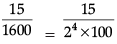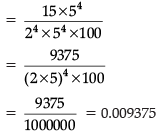So, assertion is incorrect:
Now, in case of Reason: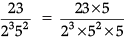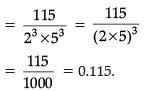So, reason is correct.

Thus, assertion is incorrect but reason is correct.

- Learn Important Definitions & Formulas of the chapter Real Numbers by going through this doc

- Test your knowledge by attempting this test on Real Numbers.

The document Assertions & Reason Type Questions: Real Numbers Notes | Study Mathematics (Maths) Class 10 - Class 10 is a part of the Class 10 Course Mathematics (Maths) Class 10.
All you need of Class 10 at this link: Class 10Use Code STAYHOME200 and get INR 200 additional OFF

## Mathematics (Maths) Class 10

58 videos|354 docs|138 tests

Track your progress, build streaks, highlight & save important lessons and more!

,

,

,

,

,

,

,

,

,

,

,

,

,

,

,

,

,

,

,

,

,

;